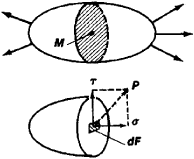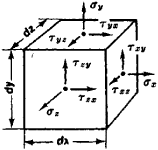Stress, Mechanical

The following article is from The Great Soviet Encyclopedia (1979). It might be outdated or ideologically biased.

Stress, Mechanical

a measure of internal forces that arise in a body being deformed as a result of external forces. When studying the stress at any point, a section is cut through the point (Figure 1). The interaction of contiguous parts of the body along the section is replaced by forces. If a force ∆P acts on the elementary area ∆S surrounding point M, the limiting value of the quotient lim (∆P/∆S) ═ is called the stress at the point M on the surface ∆S; it is a vector quantity. The components of the vector of stress are the normal stress σ along the normal to the section and the tangential stress τ, in which case 2 ═ σ2 + τ2. The totality of vectors of stress for all sections cut through point M characterizes the stressed state at the point. The stressed state is completely defined by the tensor of stresses, whose components σx, σY, σZ, τxy ═ τyx, τyz ═ τzy, and τzxτxz are precisely the stresses on the faces of an infinitely small parallelipiped formed in the vicinity of the given point (Figure 2).Figure 1Figure 2

The relationship between stress and deformations is described by relationships of elasticity theory within the elasticity limits of the material. Equations of plasticity theory apply in the elastoplastic state. Experimental studies of stress are conducted using the method of tensometry (strain measurement), as well as optical methods (for example, the optical polarization method of stress study).